# 网络科学模型¶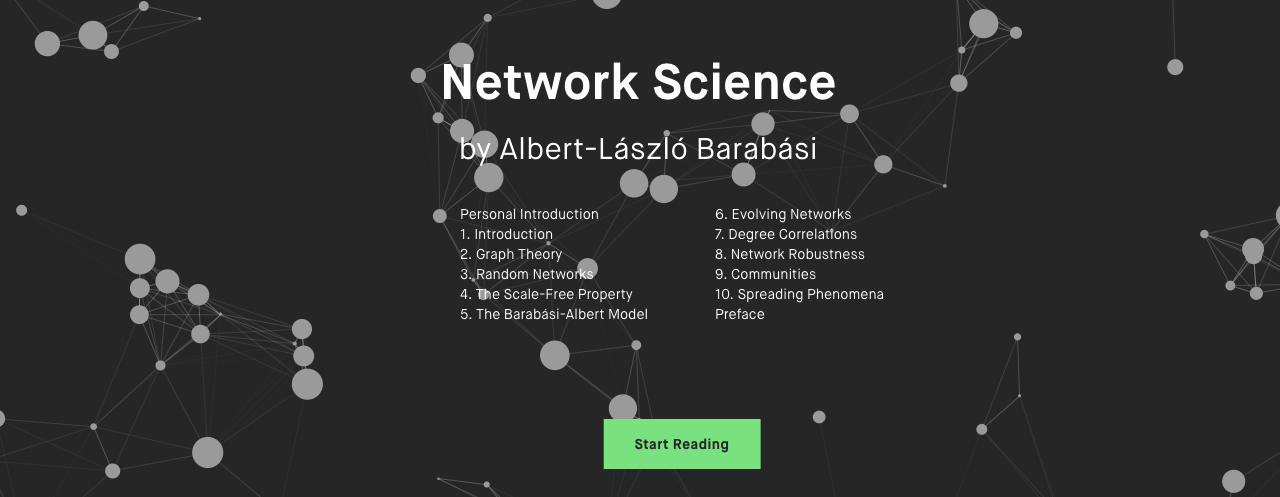The power of network science, the beauty of network visualization. Network Science, a textbook for network science, is freely available under the Creative Commons license. http://networksciencebook.com/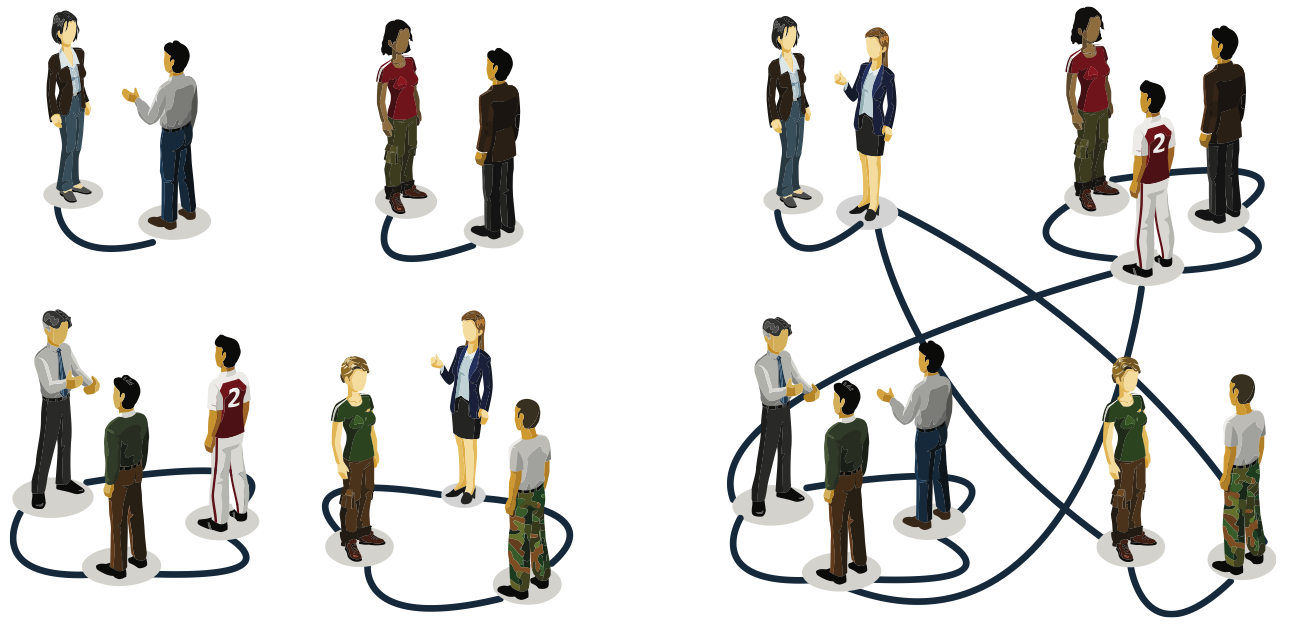## The random network model¶

### Erdös-Rényi model (1960)¶

Definition: A random graph is a graph of N nodes where each pair of nodes is connected by probability p.

### G(N, L) Model¶

N lableled nodes are connected with L randomly placed links. Erdös-Rényi(1959)

### G(N, p) Model¶

Each pair of N labeled nodes is connected with probability p. Gilbert (1959)

### To construct a random network:¶

1. Start with $N$ isolated nodes.

1. Select a node pair and generate a random number between 0 and 1. If the number exceeds $p$, connect the selected node pair with a link, otherwise leave them disconnected.

1. Repeat step (2) for each of the $\frac{N(N-1)}{2}$ node pairs.

The probability that a random network has exactly $L$ links and $N$ nodes:

$$p_L = C_{\frac{N(N-1)}{2}}^L p^L (1-p)^{\frac{N(N-1)}{2} -L}$$

### BINOMIAL DISTRIBUTION: MEAN AND VARIANCE¶

If we toss a fair coin N times, tails and heads occur with the same probability p = 1/2. The binomial distribution provides the probability $p_x$ that we obtain exactly x heads in a sequence of N throws. In general, the binomial distribution describes the number of successes in N independent experiments with two possible outcomes, in which the probability of one outcome is p, and of the other is 1-p.

$$p_x = \sum_{x}^N p^x (1-p)^{N-x}$$

The mean of the distribution (first moment) is

$$= \sum_{x = 0}^N xp_x = Np$$

$P_L$is a binomial distribution, the expected number of links in a random graph is

$$= \sum_{L = 0}^{\frac{N(N-1)}{2}} L p_L = \frac{N(N-1)}{2} p$$

$$L_{max} = \frac{N(N-1)}{2}$$

The average degree of a random network is :

$$= \frac{2}{N} = (N-1)p$$

$$k_{max} = N-1$$

### In summary¶

• the number of links in a random network varies between realizations.

• Its expected value is determined by N and p.

• If we increase p a random network becomes denser:

• The average number of links increase linearly from = 0 to Lmax

• and the average degree of a node increases from = 0 to = N-1.

### Degree Distribution¶

In a given realization of a random network some nodes gain numerous links, while others acquire only a few or no links. These differences are captured by the degree distribution, $p_k$.

$p_k$ is the probability that a randomly chosen node has degree $k$.

In a random network the probability that node i has exactly $k$ links is the product of three terms:

$k_{max} = N-1$, 节点i的边的最大数量是N-1

• The probability of node i having k links, or $p^k$.

• The probability that the remaining (N-1-k) links are missing, or $(1-p)^{N-1-k}$

• The number of ways we can select $k$ links from $N- 1$ potential links a node can have, or $C_{N-1}^k$

Consequently the degree distribution of a random network is:

$p_k = C_{N-1}^k p^k (1-p)^{N-1-k}$

which follows the binomial distribution. The shape of this distribution depends on the system size $N$ and the probability $p$.

### POISSON DISTRIBUTION¶

• Most real networks are sparse, meaning that for them $$≪ N. • the degree distribution is well approximated by the Poisson distribution p_k = e^{-} \frac{^k}{k!} 大于平均度的节点数量随着度的增加指数下降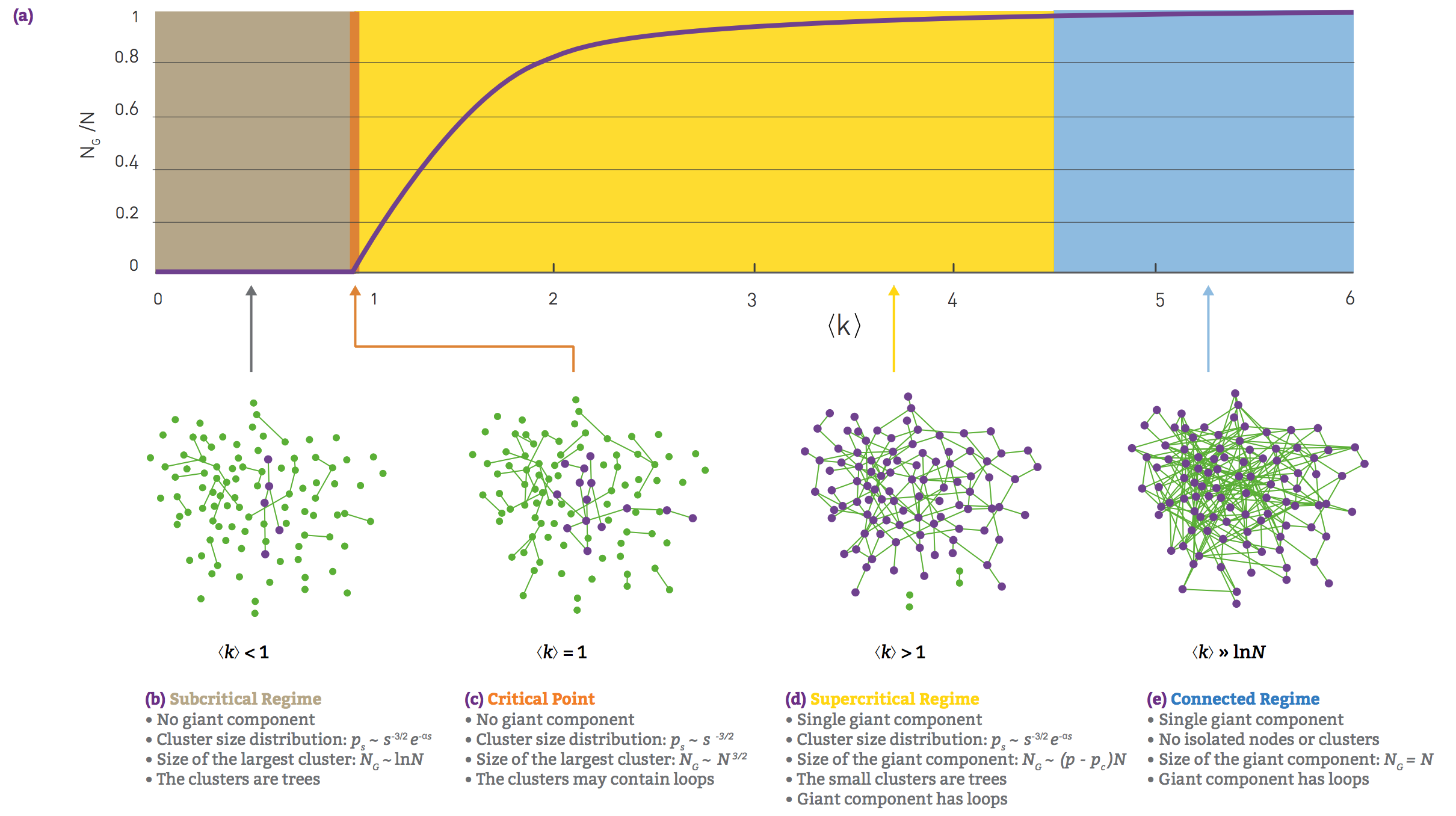The evolution of random networks The relative size of the giant component in function of the average degree$$ in the Erdős-Rényi model. The figure illustrates the phase tranisition at $= 1$, responsible for the emergence of a giant component with nonzero $N_G$. REAL NETWORKS ARE SUPERCRITICAL

## The small world phenomenon 小世界现象¶

also known as six degrees of separation, has long fascinated the general public. It states that if you choose any two individuals anywhere on Earth, you will find a path of at most six acquaintances between them.

• What does short (or small) mean, i.e. short compared to what?

• How do we explain the existence of these short distances?

Consider a random network with average degree $$. A node i in this network has on average: •$$ nodes at distance one (d=1).

• $^2$ nodes at distance two (d=2).

• $^3$ nodes at distance three (d =3).

• $^d$ nodes at distance d.• 从这个节点i走一步，到达他/她的$$个朋友 • 从节点i走两步，先到他/她的$$个朋友，再到每个朋友的$$个朋友。 • 。。。 for  ≈ 1,000, which is the estimated number of acquaintences an individual has, we expect 10^6 individuals at distance two and about a billion, i.e. almost the whole earth’s population, at distance three from us. 从一个开始的节点出发走d步，共有节点数量： N(d) = 1 + + ^2 + ^3 + …+ ^d = \frac{^{d+1}-1}{-1} ### 随机网络的直径¶ The expected number of nodes up to distance d from our starting node is N(d) N(d)比N小，当d = d_{max}的时候： N(d_{max}) \sim N 我们知道： N(d) = 1 + + ^2 + ^3 + …+ ^d = \frac{^{d+1}-1}{-1} 若$$ 远大于1，那么: $N(d_{max}) = ^{d_{max}} \sim N$，

$$d_{max} = \frac{ln N}{ln }$$

The diameter depends logarithmically on the system size.

The $\frac{1}{ln }$ term implies that the denser the network, the smaller is the distance between the nodes.

Let us illustrate the implications of (3.19) for social networks. Using $N ≈ 7 ×10^9$ and $≈ 10^3$, we obtain

$$d_{max} = \frac{ln 7 ×10^9}{ln 10^3} = 3.28$$

I. de Sola Pool and M. Kochen. Contacts and Influence. Social Networks, 1: 5-51, 1978.

### SIX DEGREES: EXPERIMENTAL CONFIRMATION¶

The first empirical study of the small world phenomena took place in 1967

• Stanley Milgram, building on the work of Pool and Kochen, designed an experiment to measure the distances in social networks.

• Milgram chose a stock broker in Boston and a divinity student in Sharon, Massachusetts as targets.

• He then randomly selected residents of Wichita and Omaha, sending them a letter containing a short summary of the study’s purpose, a photograph, the name, address and information about the target person.

• They were asked to forward the letter to a friend, relative or acquantance who is most likely to know the target person.

He found that the median number of intermediates was 5.2,

Using Facebook’s social graph of May 2011, consisting of 721 million active users and 68 billion symmetric friendship links, researchers found an average distance 4.74 between the users.

• Therefore, the study detected only ‘four degrees of separation’, closer to the prediction of than to Milgram’s six degrees.

L. Backstrom, P. Boldi, M. Rosa, J. Ugander, and S. Vigna. Four degrees of separation. In ACM Web Science 2012: Conference Proceedings, pages 45−54. ACM Press, 2012.### 大的聚集系数与小的网络直径如何并存？¶

• 1D: For a one-dimensional lattice (a line of length N) the diameter and the average path length scale linearly with $N: dmax\sim \sim N$.

• 2D: For a square lattice $dmax \sim \sim N^{1/2}$.

• 3D: For a cubic lattice $dmax \sim \sim N^{1/3}$.

• dD: In general, for a d-dimensional lattice $dmax \sim \sim N^{1/d}$.

In lattices the path lengths are significantly longer than in a random network.### 邻居彼此认识吗？¶

Local clustering coefficient

We need to estimate the expected number of links $L_i$ between the node’s $k_i$ neighbors.

In a random network the probability that two of i’s neighbors link to each other is $p$.

As there are $\frac{k_i(k_i - 1)}{2}$ possible links between the $k_i$ neighbors of node i, the expected value of $L_i$ is

$$= p\frac{k_i(k_i - 1)}{2}$$

### 局部聚集系数¶

$$<C_i> = \frac{}{\frac{1}{2} k_i(k_i - 1)} = p = \frac{}{N}$$

• For fixed $$, the larger the network, the smaller is a node’s clustering coefficient. • The local clustering coefficient of a node is independent of the node’s degree.## WS模型 （1998）¶ • Small World Property In real networks the average distance between two nodes depends lithmically on N • High Clustering The average clustering coefficient of real networks is much higher than expected for a random network of similar N and L • a regular lattice has high clustering but lacks the small-world phenomenon. • and a random network has low clustering, but displays the small-world property. Numerical simulations indicate that for a range of rewiring parameters the model’s average path length is low but the clustering coefficient is high. D. J. Watts and S. H. Strogatz. Collective dynamics of ‘small-world’ networks. Nature, 393: 409–10, 1998. • The dependence of the average path length d(p) and clustering coefficient <C(p)> on the rewiring parameter p. • Note that d(p) and <C(p)> have been normalized by d(0) and <C(0)> obtained for a regular lattice. • The rapid drop in d(p) signals the onset of the small-world phenomenon. • During this drop, <C(p)> remains high. • Hence in the range 0.001<p<0.1 short path lengths and high clustering co-exist in the network. D. J. Watts and S. H. Strogatz. Collective dynamics of ‘small-world’ networks. Nature, 393: 409–10, 1998.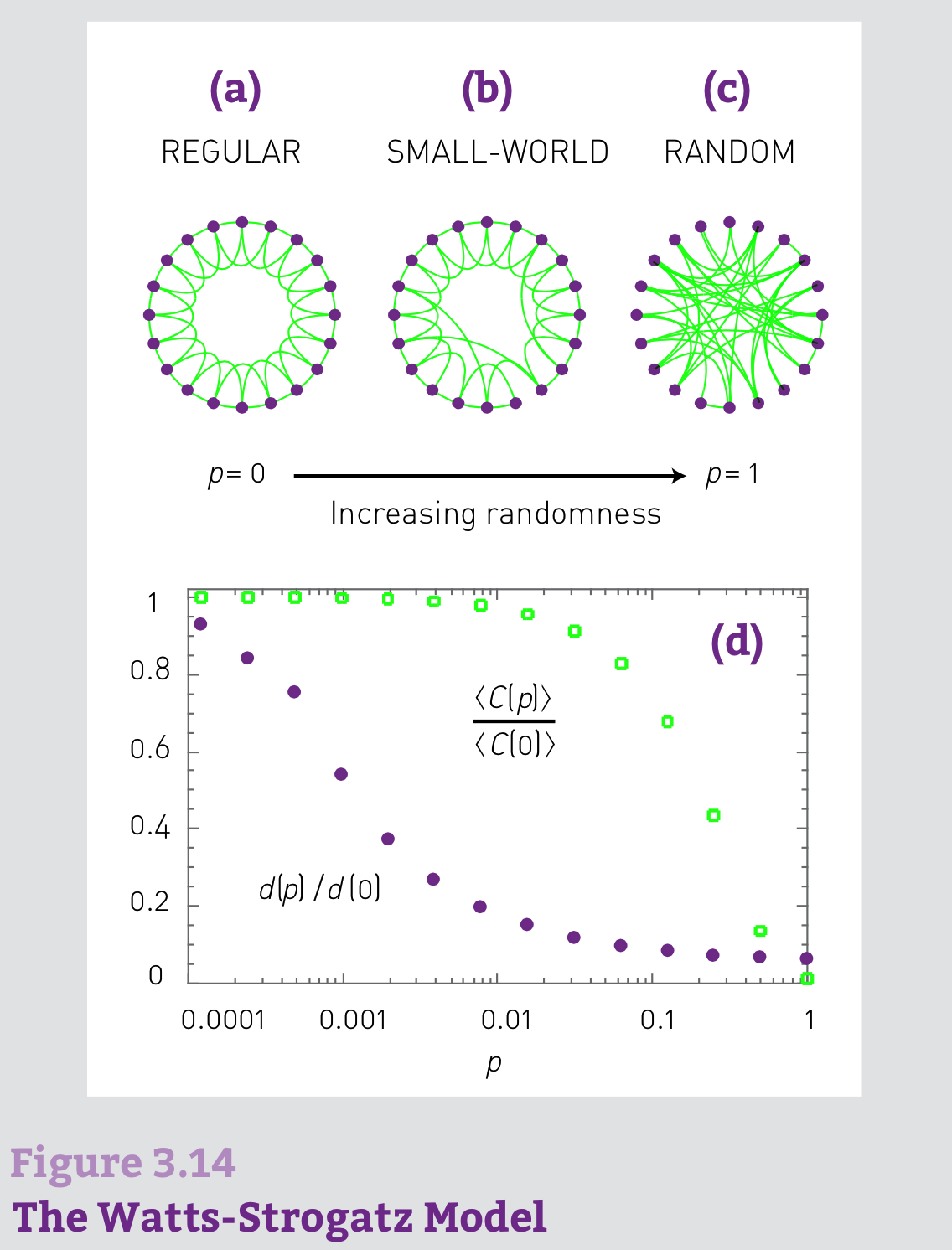### In summary¶ • we find that the random network model does not capture the clustering of real networks. • Instead real networks have a much higher clustering coefficient than expected for a random network of similar N and L. • An extension of the random network model proposed by Watts and Strogatz  addresses the coexistence of high and the small world property. • It fails to explain, however, why high-degree nodes have a smaller clustering coefficient than low-degree nodes.REAL NETWORKS ARE NOT POISSON ## BA模型 （1999）¶ Barabasi (1999) Emergence of scaling in random networks.Science-509-12 ### Scaling-Free¶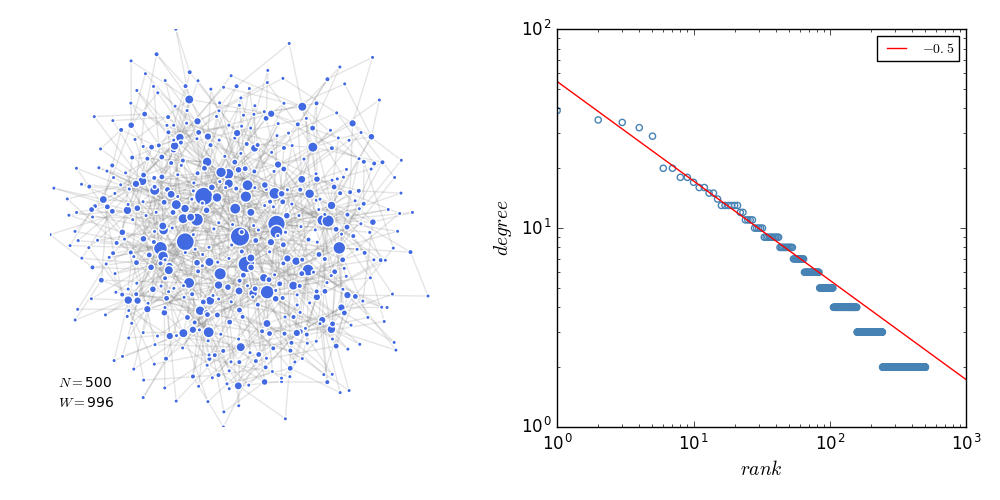The Preferential Attachment Model ### 无标度的意义¶ 度分布的n阶矩被定义为：  <k^n> = \sum_{k_{min}}^{k_{max}}k^np_k = \int_{k_{min}}^{k_{max}}k^np_kdk  （1） 低阶矩具有明确的统计意义： • n = 1的时候，一阶矩是<k^{}>，即平均度。 • n = 2的时候，二阶矩是<k^2>，可以帮助计算方差  \delta^2 = <k^2> - <k^{}>^{2} ，测量了度的离散程度（the spread in the degrees）。 • n = 3的时候，三阶矩是<k^3>, 决定了度分布的偏度（skewness），测量了p_k围绕着$$的对称性。

$p(k) = Ck^{-\gamma}$ （2）

$<k^n> = \int_{k_{min}}^{k_{max}}k^np_kdk = C \frac{k_{max}^{n- \gamma +1} - k_{min}^{n - \gamma +1}}{n - \gamma + 1}$ （3）

• 当$n - \gamma +1 <= 0$时，随着$k_{max}$增加，$k_{max}^{n- \gamma +1} \rightarrow 0$。所有满足$n <= \gamma -1$的n阶矩都是有限的。

## 参考文献¶

• Barabasi 2016 Network Science. Cambridge http://networksciencebook.com/

• Barabasi (1999) Emergence of scaling in random networks.Science-509-12.pdf

• Barabasi (1999) Mean-field theory for scale-free random networks. PA.pdf

• Albert & Barabasi (2002) Statistical mechanics of complex networks. RMP.pdf

• Principle of Locality I: Hacking the Continuum Mean-Field Technique in Network Modeling http://www.jianshu.com/p/97f674267d3e